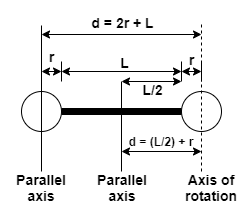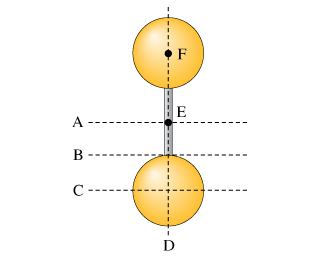# Problem: Two identical uniform solid spheres are attached by a solid uniform thin rod, as shown in the figure. There lies on a line connecting the centers of mass of the two spheres. The axes A, B, C, and D are in the plane of the page(which also contains the centers of mass of the spheres and the rod), while axes E and F (represented by black dots) are perpendicular to the page.Rank the moments of inertia of this object about the axes indicated.Rank from largest to smallest. To rank items as equivalent, overlap them.A,B,C,D,E,F

###### FREE Expert Solution

Moment of inertia of sphere,

$\overline{){\mathbf{I}}{\mathbf{=}}\frac{\mathbf{2}}{\mathbf{5}}{\mathbf{m}}{{\mathbf{r}}}^{{\mathbf{2}}}}$

Moment of inertia of solid cylinder,

$\overline{){\mathbf{I}}{\mathbf{=}}\frac{\mathbf{1}}{\mathbf{2}}{\mathbf{m}}{{\mathbf{r}}}^{{\mathbf{2}}}}$

Moment of inertia of a rod about the center,

$\overline{){\mathbf{I}}{\mathbf{=}}\frac{\mathbf{1}}{\mathbf{12}}{{\mathbf{ML}}}^{{\mathbf{2}}}}$

Parallel axis theorem,

The image below illustrates the parallel axis theorem. The image shows all the dimensions necessary in this problem but does not include all the axes.Let the top sphere be 1 and the lower sphere 2. Mass of sphere is m, the mass of the rod is M, length of the rod L, and the radius of the rod is R.

The rod is rotating about its center of mass, and the spheres are each a distance d = (r + L/2) from the axis of rotation.

Spere 1 - top sphere:

The sphere is rotating on a parallel axis. Its moment of inertia is the sum of I(center of mass of sphere) and  Md2,

d = (r + L/2)

I1 = (2/5)mr2 + m(r + L/2)2

Sphere 2 - lower sphere:

The sphere is rotating on a parallel axis. Its moment of inertia is the sum of I(center of mass of sphere) and  Md2,

d = (r + L/2)

I2 = (2/5)mr2 + m(r + L/2)2

Connecting rod:

The rod is rotating through its center of mass. It has no parallel axis component.

Ir = (1/12)ML2

IA = I1 + I2 + Ir = (2/5)mr2 + m(r + L/2)2 + (2/5)mr2 + m(r + L/2)2 + (1/12)ML2

87% (237 ratings)###### Problem Details

Two identical uniform solid spheres are attached by a solid uniform thin rod, as shown in the figure. There lies on a line connecting the centers of mass of the two spheres. The axes A, B, C, and D are in the plane of the page(which also contains the centers of mass of the spheres and the rod), while axes E and F (represented by black dots) are perpendicular to the page.Rank the moments of inertia of this object about the axes indicated.

Rank from largest to smallest. To rank items as equivalent, overlap them.

A,B,C,D,E,F